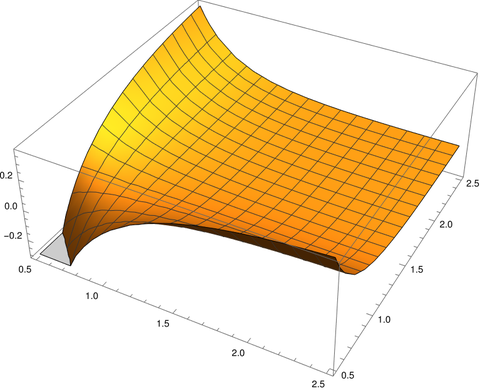# Upper and lower bounds on the beta function

The beta function B(x, y) is defined byand is the normalizing constant for the beta probability distribution. It is related to the gamma function viaThe beta function comes up often in applications. It can be challenging to work with, however, and so estimates for the function are welcome.

The function 1/xy gives simple approximation and upper bound for B(x, y). Alzer  proved that when x > 1 and y > 1where the constant b is defined byCerone  gives a different bound which varies with x and y and is usually better than Alzer’s bound. For x and y greater than 1, Cerone showswhereandCerone’s bound is slightly larger in the worst case, near x = y = (3 + √5)/2, but is smaller in general.

The difference between B(x, y) and 1/xy is largest when x or y is small. We can visualize this with the Mathematica command

    Plot3D[Beta[x, y] - 1/(x y), {x, 0.5, 2.5}, {y, 0.5, 2.5}]

which produces the following plot.The plot dips down in the corner where x and y are near 0.5 and curls upward on the edges where one of the variables is near 0.5 and the other is not.

Let’s look at B(x, y) and 1/xy at along a diagonal slice (3t, 4t).This suggests that approximating B(x, y) with 1/xy works best when the arguments are either small or large, with the maximum difference being when the arguments are moderate-sized. In the plot we see B(3, 4) is not particularly close to 1/12.

Next lets look at 1/xyB(x, y) along the same diagonal slice.This shows that the error bound C(x) C(y) is not too tight, but better than the constant bound except near the maximum of 1/xyB(x, y).

## Related posts

 H. Alzer. Monotonicity properties of the Hurwitz zeta function. Canadian Mathematical Bulletin 48 (2005), 333–339.

 P. Cerone. Special functions: approximations and bounds. Applicable Analysis and Discrete Mathematics, 2007, Vol. 1, No. 1, pp. 72–91